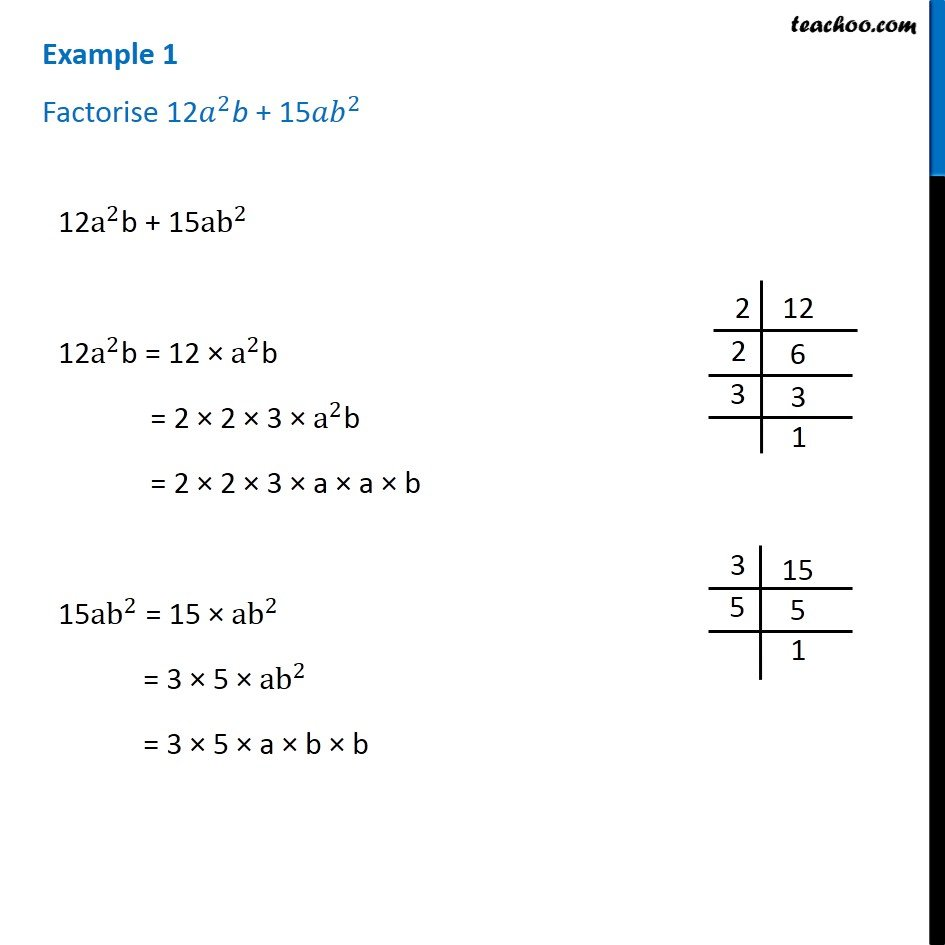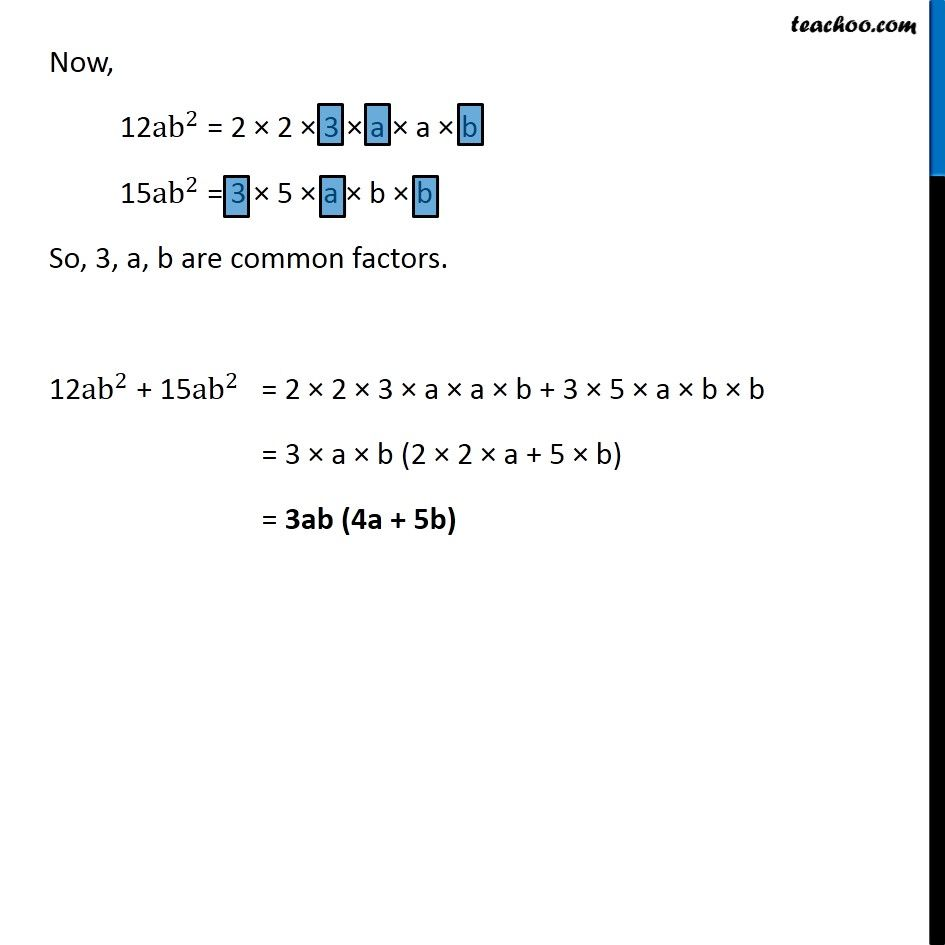Subscribe to our Youtube Channel - https://you.tube/teachoo

1. Chapter 14 Class 8 Factorisation
2. Serial order wise
3. Examples

Transcript

Example 1 Factorise 12𝑎^2b + 15〖𝑎𝑏〗^2 12a^2b + 15ab^2 12a^2b = 12 × a^2b = 2 × 2 × 3 × a^2b = 2 × 2 × 3 × a × a × b 15ab^2 = 15 × ab^2 = 3 × 5 × ab^2 = 3 × 5 × a × b × b Now, 12ab^2 = 2 × 2 × 3 × a × a × b 15ab^2 = 3 × 5 × a × b × b So, 3, a, b are common factors. 12ab^2 + 15ab^2 = 2 × 2 × 3 × a × a × b + 3 × 5 × a × b × b = 3 × a × b (2 × 2 × a + 5 × b) = 3ab (4a + 5b)

Examples